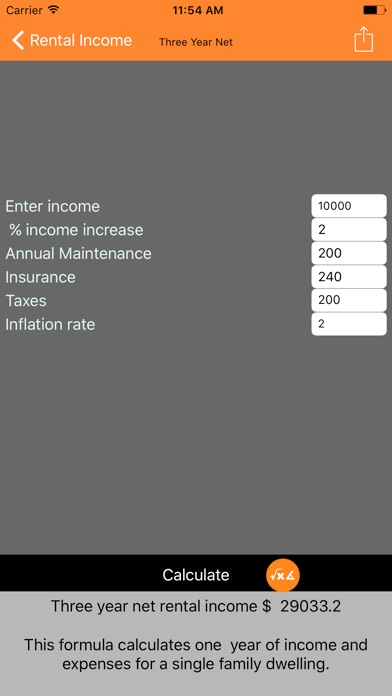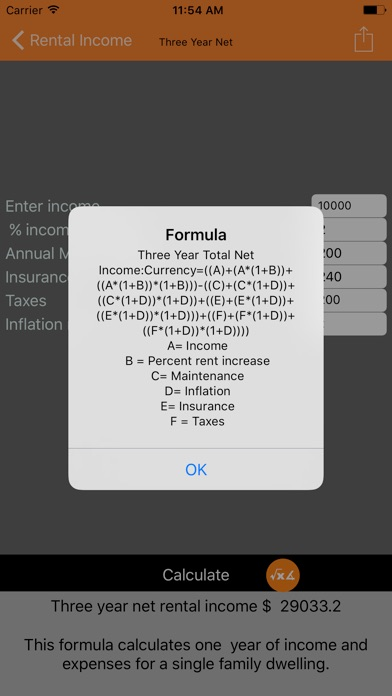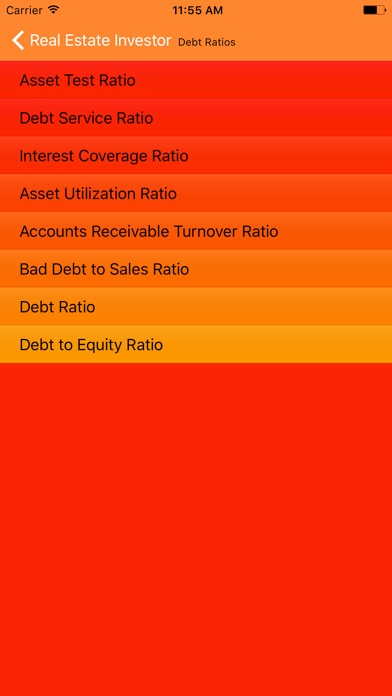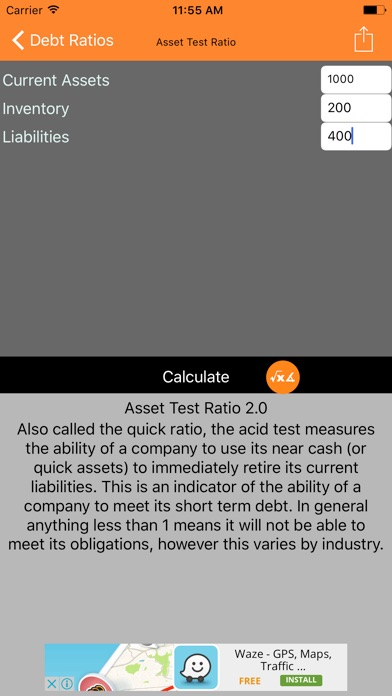# Real Estate Investor

## iOS UniversalFinance

\$1.99

REAL ESTATE INVESTOR is an important tool for any real estate investor. Whether you are investing in a two family home, a large apartment house or commercial property this application can calculate valuable information for you. Sections include: Capitalization, Debt Ratios, Depreciation, Interest, Investment Returns, Mortgages, Rent Multipliers, Valuation and Rental Income. Whether you want to calculate the market value property, vacancy loss, rent multiplier or total net operating income Real Estate Investor is the app for you.

A total of 60 formulas are included in this version of the product. If you are looking for a formulas that is not currently included in the program just e-mail us and we will try to add it in a future update.

REAL ESTATE INVESTOR includes the following formulas:
• Capitalization Rate
• Capitalization Rate
• Value
• Net Income Multiplier
• Net Income Multiplier

DEBT RATIOS
• Acid Test
• Debt Service Ratios
• Interest Coverage
• Asset Utilization
• Accounts Receivables Turnover Ratios
• Bad Debt To Sales Ratios
• Debt Ratio
• Debt To Equity

DEPRECIATION
• Depreciation-Yearly S.L
• Declining Value Amount
• Double Declining Value
• Value After Years

INTEREST FORMULAS
• Future Value
• Daily Interest
• Interest Computation
• Days Of Savings
• Annual Percentage Rate
• A.P.R Installment Loan

INVESTMENTS
• Discounted Value Of Note
• Holding Period Return
• Capitalization Rates
• Equivalent Taxable Yield
• Rule 72’s
• Number Of Years To Double Investment

MORTGAGE
• Monthly Mortgage Payment
• Total Monthly Carrying Cost
• Monthly Points Mortgage Payment

RENT MULTIPLIER
• Gross Rent Multiplier
• Market Value
• Gross Rent Multiplier

RENTAL INCOME
• Single Family Home
• Total Net Income
• Three Year Gross Income
• Three Year Total Net Income
• Gross Potential Income
• One Year Income 2-3 Family House
• Three Year Income 2-3 Family House
• Apartment House
• 3 Year Apartment House
• Commercial Rent Income
• Vacancy and Credit Loss
• Credit/Vacancy Allowance
• Effective Gross Income
• Gross Operating Income
• Net Operating Income
• Total Net Operating Income

VALUATIONS
• Price, Income, Expenses Per Unit
• Price Per Unit
• Income Per Unit
• Expenses Per Unit
• Price, Income, Expenses Per Square Foot
• Price Per Square Foot
• Income Per Square Foot
• Expenses Per Square Foot

••••